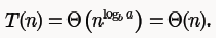Courses

# Test: Recurrence & Searching- 1

## 10 Questions MCQ Test RRB JE for Computer Science Engineering | Test: Recurrence & Searching- 1

Description
This mock test of Test: Recurrence & Searching- 1 for Computer Science Engineering (CSE) helps you for every Computer Science Engineering (CSE) entrance exam. This contains 10 Multiple Choice Questions for Computer Science Engineering (CSE) Test: Recurrence & Searching- 1 (mcq) to study with solutions a complete question bank. The solved questions answers in this Test: Recurrence & Searching- 1 quiz give you a good mix of easy questions and tough questions. Computer Science Engineering (CSE) students definitely take this Test: Recurrence & Searching- 1 exercise for a better result in the exam. You can find other Test: Recurrence & Searching- 1 extra questions, long questions & short questions for Computer Science Engineering (CSE) on EduRev as well by searching above.
*Answer can only contain numeric values
QUESTION: 1

### The given diagram shows the flowchart for a recursive function  A(n). Assume that all statements, except for the recursive calls, have O(1) time complexity. If the worst case time complexity of this function is O(nα) then the least possible value (accurate up to two decimal positions) of α is ________. Flow chart for Recursive Function A(n).Solution:

If they are asking for worst case complexity hence,

By calling A(n) we get A(n/2) 5 times,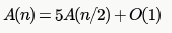Hence by applying masters theorem,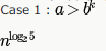Thus value of alpha will be 2.32

QUESTION: 2

### The recurrence relation that arises in relation with the complexity of binary search is:

Solution:

It is B. searching for only one half of the list. leading to T(n/2) + constant time in comparing and finding mid element.

QUESTION: 3

### The recurrence relation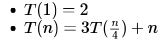has the solution T (n) equal to

Solution:

According to Master theorem,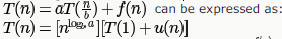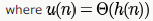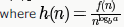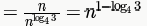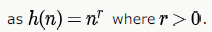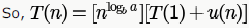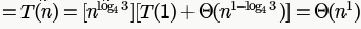QUESTION: 4

Let

T(n) be the function defined by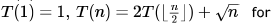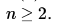Which of the following statements is true?

Solution:

using master method (case 1)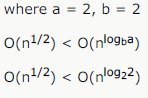QUESTION: 5

The solution to the recurrence equation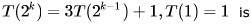Solution: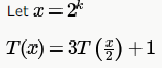We can apply Master's theorem case 1 with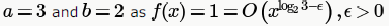So,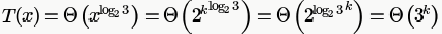So, only option possible is B.

We can also directly solve as follows: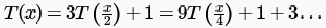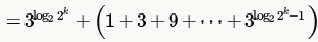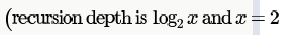OR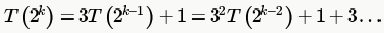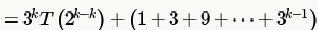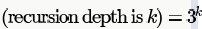QUESTION: 6

The running time of the following algorithm

Procedure A(n)

If n<=2 return (1) else return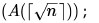is best described by:

Solution:

The complexity will be the number of times the recursion happens which is equal to the number of times we can take square root of n recursively, till n becomes 2.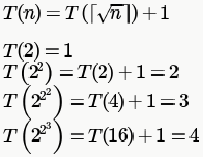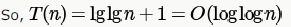QUESTION: 7

Consider the following recurrence relation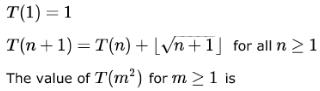Solution: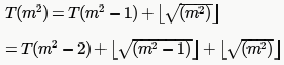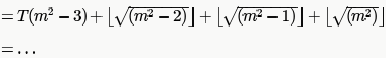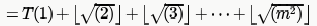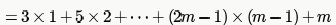(We are taking floor of square root of numbers, and between
successive square roots number of numbers are in the series 3,5,7... like 3 numbers from 1...4,5numbers from 5-9 and so on).

We can try out options here or solve as shown at end:

PutQUESTION: 8

The recurrence equation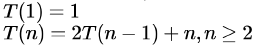evaluates to

Solution: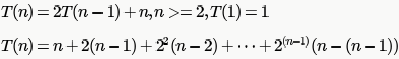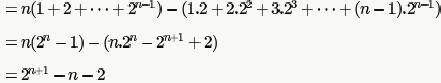QUESTION: 9

Consider a list of recursive algorithms and a list of recurrence relations as shown below. Each recurrence relation corresponds to exactly one algorithm and is used to derive the time complexity of the algorithm.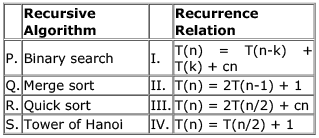Which of the following is the correct match between the algorithms and their recurrence relations?

Solution:

These are examples of some standard algorithms whose
Merge Sort:    T(n) = 2T(n/2) + Θ(n). It falls in case 2 as c is 1 and Logba] is also 1 and  the solution is Θ(n Logn) //time complexity can be evaluated using Master Method

Binary Search: T(n) = T(n/2) + Θ(1). It also falls in case 2 as c is 0 and Logba is also 0 and the solution is Θ(Logn) //time complexity can be evaluated using Master Method

Quick Sort : Time taken by QuickSort in general can be written as  T(n) = T(k) + T(n-k-1) +(n)

Tower of Hanoi : T(n) = 2T(n-1) + 1

QUESTION: 10

Let T(N) be a function defined by the recurrence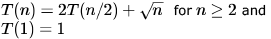Which of the following statements is TRUE?

Solution:

Option C it can be done by Master theorem.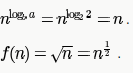So,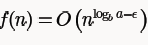is true for any real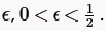Hence Master theorem Case 1 satisfied,